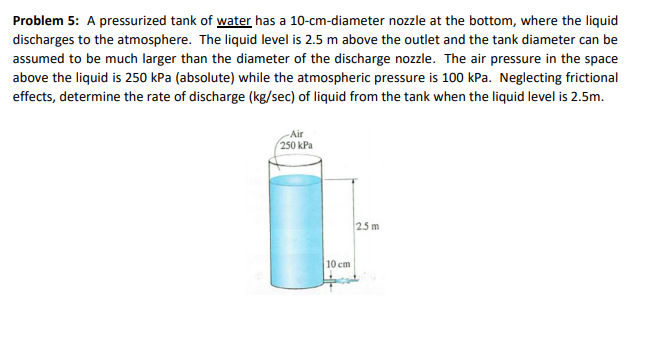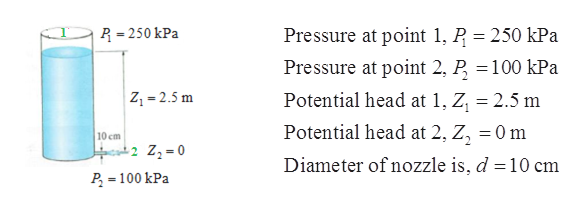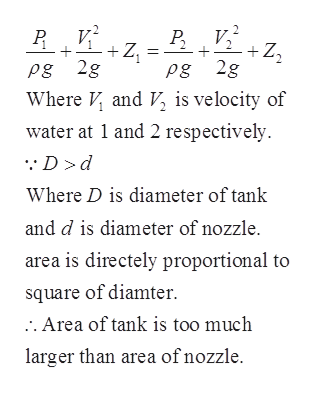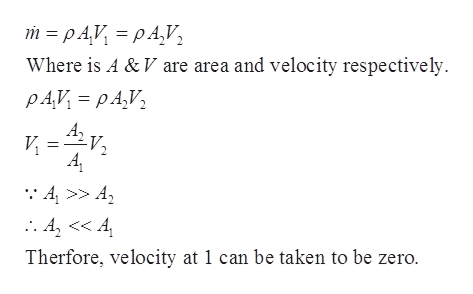# Problem 5: A pressurized tank of water has a 10-cm-diameter nozzle at the bottom, where the liquiddischarges to the atmosphere. The liquid level is 2.5 m above the outlet and the tank diameter can beassumed to be much larger than the diameter of the discharge nozzle. The air pressure in the spaceabove the liquid is 250 kPa (absolute) while the atmospheric pressure is 100 kPa. Neglecting frictionaleffects, determine the rate of discharge (kg/sec) of liquid from the tank when the liquid level is 2.5mAir250 kPa25m10 cm

Question
6 viewshelp_outlineImage TranscriptioncloseProblem 5: A pressurized tank of water has a 10-cm-diameter nozzle at the bottom, where the liquid discharges to the atmosphere. The liquid level is 2.5 m above the outlet and the tank diameter can be assumed to be much larger than the diameter of the discharge nozzle. The air pressure in the space above the liquid is 250 kPa (absolute) while the atmospheric pressure is 100 kPa. Neglecting frictional effects, determine the rate of discharge (kg/sec) of liquid from the tank when the liquid level is 2.5m Air 250 kPa 25m 10 cm fullscreen
check_circle

Step 1

Taking point 1 above liquid surface and point 2 just after nozzle exit.help_outlineImage TranscriptioncloseR 250 kPa Pressure at point 1, P 250 kPa Pressure at point 2, P 100 kPa Potential head at 1, Z 2.5 m Z1-2.5m Potential head at 2, Z, = 0 m 10 cm 2 Z2 0 Diameter of nozzle is, d 10 cm P 100 kPa = fullscreen
Step 2

The principle of conservation of energy in fluid flow is given by Bernoulli’s equation. Bernoulli’s equation can be applied between two points for the same streamlines for inviscid flow. Hence, applying Bernoulli’s equation between 1 & 2.help_outlineImage TranscriptioncloseР. +Z Pg 2g Where and V is velocity of water at 1 and 2 respectively V +Z2 Pg 2g Dd Where D is diameter of tank and d is diameter of nozzle area is directely proportional to square of diamter . Area of tank is too much larger than area ofnozzle fullscreen
Step 3

From conservation of m...help_outlineImage Transcriptionclosem pAV pA,V Where is A & V are area and velocity respectively PAV pA,V :4>> A Therfore, velocity at 1 can be taken to be zero. fullscreen

### Want to see the full answer?

See Solution

#### Want to see this answer and more?

Solutions are written by subject experts who are available 24/7. Questions are typically answered within 1 hour.*

See Solution
*Response times may vary by subject and question.
Tagged in

### Fluid Mechanics